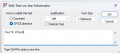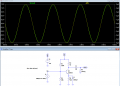# one transistor amplifier circuit

Joined Jun 16, 2023
128
hi

I am reading an electronics book by Thomas Floyd. I am simulating a simple ce amp circuit from his text (see attched).
I have 2 questions:

1. The sim says Vout is 1.166 volts but the plot has a + peak of 1.156 volts and a - peak of 1.360 volts. So it is skewed.
Why would this be?

2. The sim results in a gain of approx 66.
calculated gain at low freq is 1k / 560 + 6.72 = -1.76
calculated gain at hi freq is 1k / 6.72 = -148.81
Why?

RS

#### Papabravo

Joined Feb 24, 2006
20,600
There could be several explanations for small deviations. These mostly come down to small differences in the modeling assumptions. With respect to gain at different frequencies you don't really expect the gain to be constant over a wide frequency range -- do you?

Joined Jun 16, 2023
128
Hi E

Not sure what you mean by " Use AC=1 for actual dB results on AC analysis. " I am new to Spice.

If I change the x-axis to "linear" it appears that I should have a Vout of 2.59 volts from 20 khz to
approx 130Khz, which would indicate a gain of 130.

If you do a transient analysis the plot is skewed. Is there no way to make it symmetrical about the x-axis?

RS

Last edited:

#### crutschow

Joined Mar 14, 2008
33,348
The sim says Vout is 1.166 volts but the plot has a + peak of 1.156 volts and a - peak of 1.360 volts. So it is skewed.
Why would this be?
Due to the distortion of the amp, which is about 6.8% at that SINE input voltage.
Reducing the input level will reduce the distortion.
The stage has high distortion since there is no negative feedback.
The distortion is due to the log (diode) relation between the base-emitter input voltage and the base input current.
Breaking the emitter resistor into a small, un-bypassed, added emitter resistor with a larger added bypassed resistor (total = 560Ω) will reduce the distortion (and, of course, also the gain).

The .four analysis directive (below) will show the distortion value in the SPICE Error Log.Last edited:

#### crutschow

Joined Mar 14, 2008
33,348
Not sure what you mean by " Use AC=1 for actual dB results on AC analysis. "
The dB reading is relative to the input voltage, so if you use 1V then the output will be the gain directly in dB.

#### LvW

Joined Jun 13, 2013
1,683
calculated gain at low freq is 1k / 560 + 6.72 = -1.76
calculated gain at hi freq is 1k / 6.72 = -148.81
Why?
RS
Please, can you explain the used gain formula - in particular the value "6.72" ?

#### crutschow

Joined Mar 14, 2008
33,348
This change in AC analysis makes it symmetrical about X axis
I believe he's referring to the asymmetry in the transient SINE analysis.

Joined Jun 16, 2023
128
Please, can you explain the used gain formula - in particular the value "6.72" ?
r'e = 25mV / IE = 25 mV / 3.72 mA = 6.72 Ω

Joined Jun 16, 2023
128
I believe he's referring to the asymmetry in the transient SINE analysis.
Yes, that is what I am referring to.

#### ericgibbs

Joined Jan 29, 2010
18,222

#### LvW

Joined Jun 13, 2013
1,683
2. The sim results in a gain of approx 66.
calculated gain at low freq is 1k / 560 + 6.72 = -1.76
calculated gain at hi freq is 1k / 6.72 = -148.81
Why?

RS
Is your question related to the calculated values only or do you want an explanation for the difference between simulation and calculation?
In your own interest: A question should be as clear as possible - and the answer as simple as possible (but not simpler!)

Last edited:

#### Audioguru again

Joined Oct 21, 2019
6,439
I agree that the original circuit has no bass response because CE is too small and has horrible but normal distortion.
A transistor normally produces distortion when its output level is fairly close to the supply voltage and it has no negative feedback. It is impossible to measure the gain when the even-harmonics distortion is so high.

#### MrAl

Joined Jun 17, 2014
10,900
hi

I am reading an electronics book by Thomas Floyd. I am simulating a simple ce amp circuit from his text (see attched).
I have 2 questions:

1. The sim says Vout is 1.166 volts but the plot has a + peak of 1.156 volts and a - peak of 1.360 volts. So it is skewed.
Why would this be?

2. The sim results in a gain of approx 66.
calculated gain at low freq is 1k / 560 + 6.72 = -1.76
calculated gain at hi freq is 1k / 6.72 = -148.81
Why?

RS
Hello there,

Single transistor CE amplifiers never produce an output that is completely void of at least a little distortion. That's because transistors are not perfectly linear devices. There are ways to improve, but still not able to get exactly what you put in.

The attachment shows that this circuit is no different. The output is compared directly with a pure sine wave. The pure sine is the red plot, the output is the green plot.
You can see that the output does not match the sine wave exactly, and that means the output will APPEAR to have some DC offset. Really it's just the way waves zero themselves when the top and bottom sections are of different widths or just different in some other way.
If the distortion was zero, the output would show a perfect centering at 0v, so if the positive peak was 1.2v then the bottom peak would be -1.2v, but for a distorted wave that usually won't happen unless the negative section is distorted in exactly the same way as the positive section is, and in this circuit that's not the case either.

#### crutschow

Joined Mar 14, 2008
33,348
Single transistor CE amplifiers never produce an output that is completely void of at least a little distortion. That's because transistors are not perfectly linear devices. There are ways to improve, but still not able to get exactly what you put in.
Interestingly, the distortion is greatly reduced if a current instead of a voltage signal source is used (as would be expected).
The circuit below has a distortion of <0.2% with no negative feedback.

So driving one stage from the collector of the previous stage with a high value collector resistor should reduce the stage distortion.Last edited:
•Danko

#### MrAl

Joined Jun 17, 2014
10,900
Interestingly, the distortion is greatly reduced if a current instead of a voltage signal source is used (as would be expected).
The circuit below has a distortion of <0.2% with no negative feedback.

So driving one stage from the collector of the previous stage with a high value collector resistor would reduce the stage distortion.

View attachment 297759
Hi Cruts,

That's very interesting and would be also interesting to test in a real-life circuit.

I would think then that the distortion comes from the small change in base emitter diode forward voltage drop. Driven with a voltage, there is an inherent offset, which in turn means the current into the base will vary in a nonlinear way. With a current input, the current would naturally be whatever it was set to be, so no influence from the diode forward voltage drop.
I think this is why we usually see an output voltage that is skinnier for the negative portion than for the top portion (CE amplifier).

If i get a chance I'll try this test with a real transistor.

Last edited:

#### Audioguru again

Joined Oct 21, 2019
6,439
The distorted circuit has a fairly high gain. I showed in post #14 that the distortion is much higher when the input level is increased.
Hifi audio circuits used transistors years ago with negative feedback to reduce the distortion.
Today, opamps are used producing distortion so low that it cannot be heard and be barely measured.

#### crutschow

Joined Mar 14, 2008
33,348
If i get a change I'll try this test with a real transistor.
That would be interesting.

#### MrAl

Joined Jun 17, 2014
10,900
That would be interesting.
Hi,

It would be easy to test with a real transistor if I didn't have to first create a constant current drive. I'd have to rig up an op amp VCCS drive i guess.